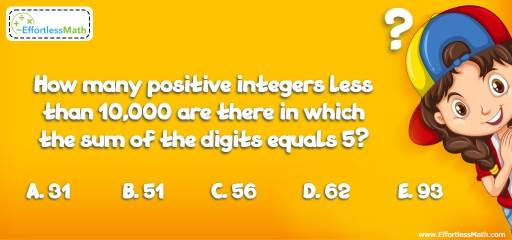# Number Properties Puzzle – Challenge 9

Do you enjoy exercising your brain? If so, then this puzzle is just for you. Let's see if you can solve this Math puzzle!## Challenge:

How many positive integers less than 10,000 are there in which the sum of the digits equals 5?

A- 31

B- 51

C- 56

D- 62

E- 93

### The Absolute Best Book to challenge your Smart Student!

We are looking for a number that the sum of its digits equals 5.
Pairs possible: $$0,0,0,5; 1,4,0,0; 0,0,2,3; 1,3,1,0; 1,2,1,1; 0,1,2,2$$
Number of options for each pair:
$$0,0,0,5 = \frac{4!}{3!} = \frac{4 ×3 ×2 ×1}{3 ×2 ×1} = 4 (5, 50, 500, 5,000)$$
$$1,4,0,0 = \frac{4!}{2!} = \frac{4 ×3 ×2 ×1}{2 ×1} = 12 (14, 41, 104, 140, 410, 401, 1004, 1040, 1400, 4001, 4010, 4100)$$
$$0,0,2,3 = \frac{4!}{2!} = \frac{4 ×3 ×2 ×1}{2 ×1} = 12 (23, 32, 230, 320, …)$$
$$1,3,1,0 = \frac{4!}{2!} =\frac{4 ×3 ×2 ×1}{2 ×1)} = 12 (113, 131, 311, 1013, …)$$
$$1,2,1,1 = \frac{4!}{3!} = \frac{4 ×3 ×2 ×1}{3 ×2 ×1} = 4 (1112, 1121, 1211, 2111)$$
$$0,1,2,2 = \frac{4!}{2! }=\frac{4 ×3 ×2 ×1}{2 ×1} = 12 (122, 212, 221, 1022, …)$$
Total: $$12 + 12 + 12 + 12 + 4 + 4 = 56$$

### What people say about "Number Properties Puzzle – Challenge 9 - Effortless Math: We Help Students Learn to LOVE Mathematics"?

No one replied yet.

X
30% OFF

Limited time only!

Save Over 30%

SAVE $5 It was$16.99 now it is \$11.99